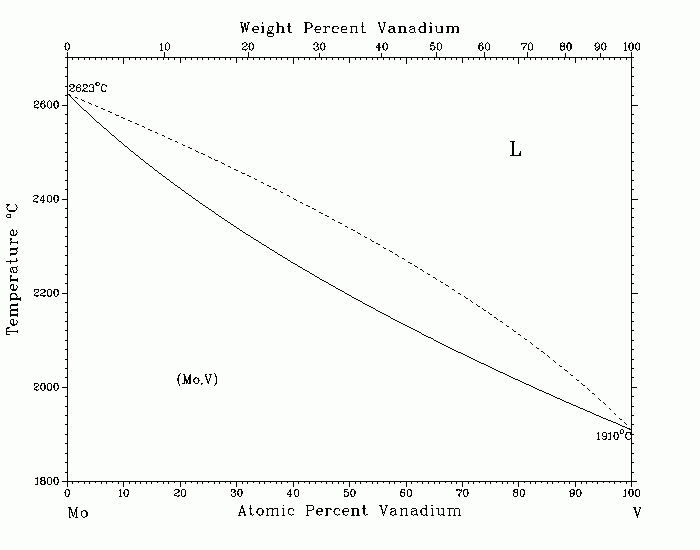# Фазовая диаграмма системы Mo-VMo-V (Molybdenum-Vanadium) J.F. Smith The Mo-V system provides an example of one simple limit for binary phase relationships-complete miscibility in both the liquid and solid states. Data of [69Rud] were used to generate the assessed, calculated phase diagram. The Miedema approach [80Deb] indicated that the interaction parameters for both solid and liquid Mo-V alloys should be near zero, and the solutions should therefore be nearly ideal. Accordingly, using or based on the Gibbs energies of fusion for Mo [83Cha] and V [81Smi], the liquidus and solidus were calculated in the ideal limit. Most of the experimental liquidus and solidus data points lie respectively above or below the ideal lines. Accordingly, interaction parameters were introduced into the calculations with regular- solution approximations. A temperature of 2300 C was selected as being near the midrange, and data of [69Rud] show that at that temperature, the mole fraction of V in the solidus is near XV = 0.355 and in the liquidus is near XV = 0.558. Two simultaneous equations were then developed from this combination of temperature and compositions, by equating the partial molar Gibbs energy of V in the solid with that of the liquid and similarly for the partial molar Gibbs energy of Mo. Solution of these equations for the interaction parameters produced values of 24 905 J/mol for the bcc solid solution and 23 151 J/mol for the liquid solution. These parameters were then used with the accepted melting temperatures of Mo and V to generate the solidus and liquidus lines shown in the assessed diagram. The crystal structure of the (Mo,V) solid solution is cI2, Im3m, A2, W type. 69Rud: E. Rudy, U.S. Air Force Rep. No. AFML-TR-65-2, Part V, 118-120 (1969). 80Deb: F.R. deBoer, R. Boom, and A.R. Miedema, Physica, 101B, 294-319 (1980). 81Smi: J.F. Smith, Bull. Alloy Phase Diagrams, 2, 40-41 and 172 (1981). 83Cha: M.W. Chase, Bull. Alloy Phase Diagrams, 4, 124 (1983). Published in Phase Diagrams of Binary Vanadium Alloys, 1989. Complete evaluation contains 3 figures, 1 table, and 16 references. 1